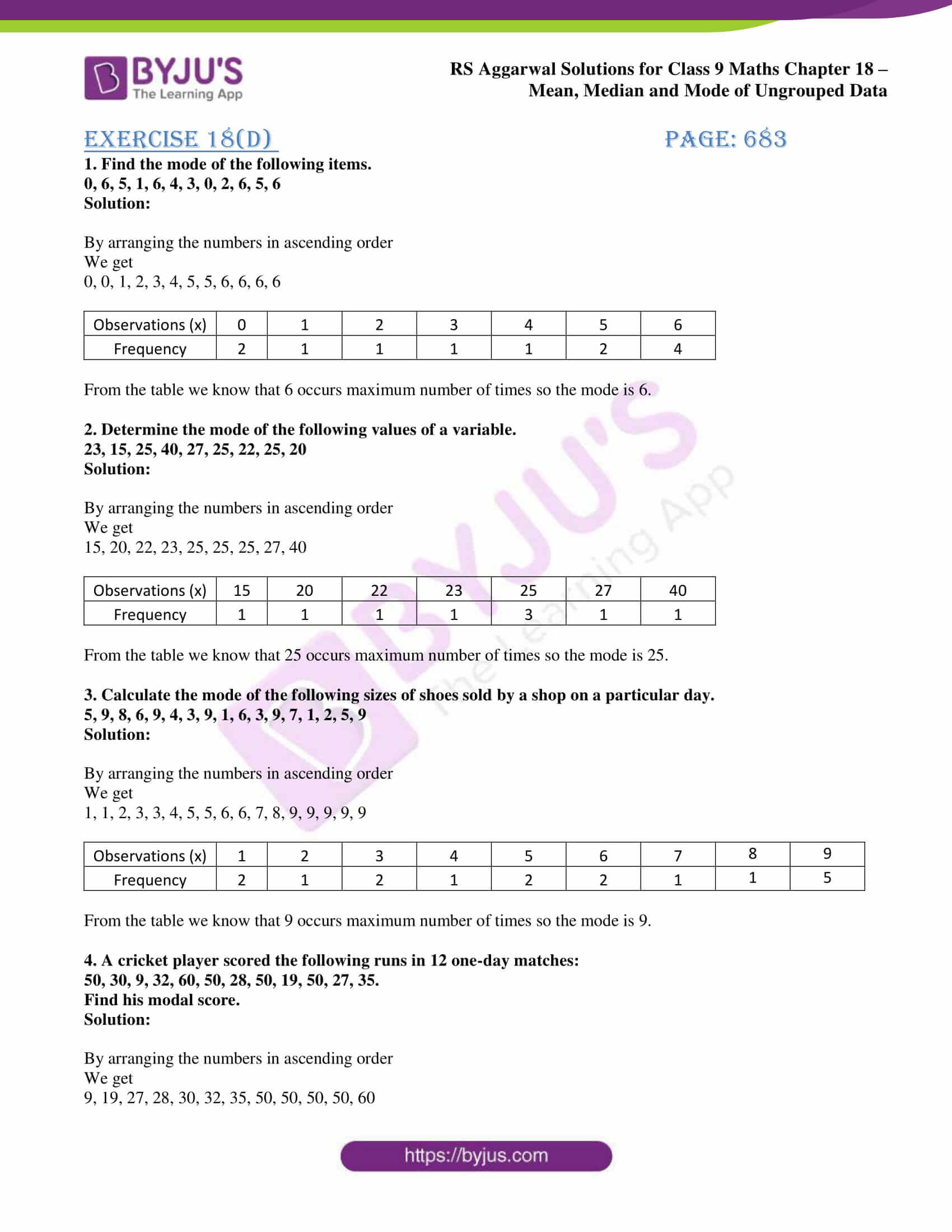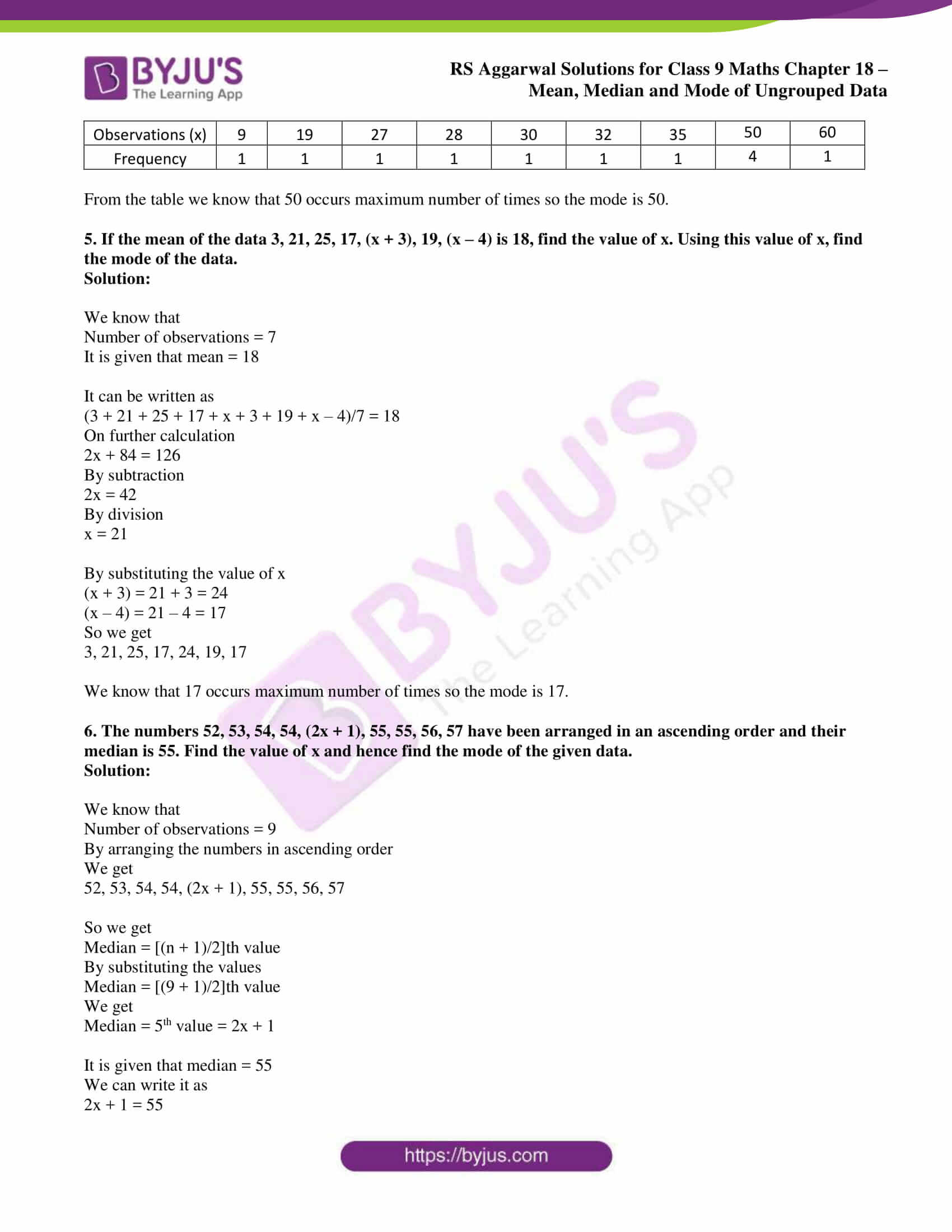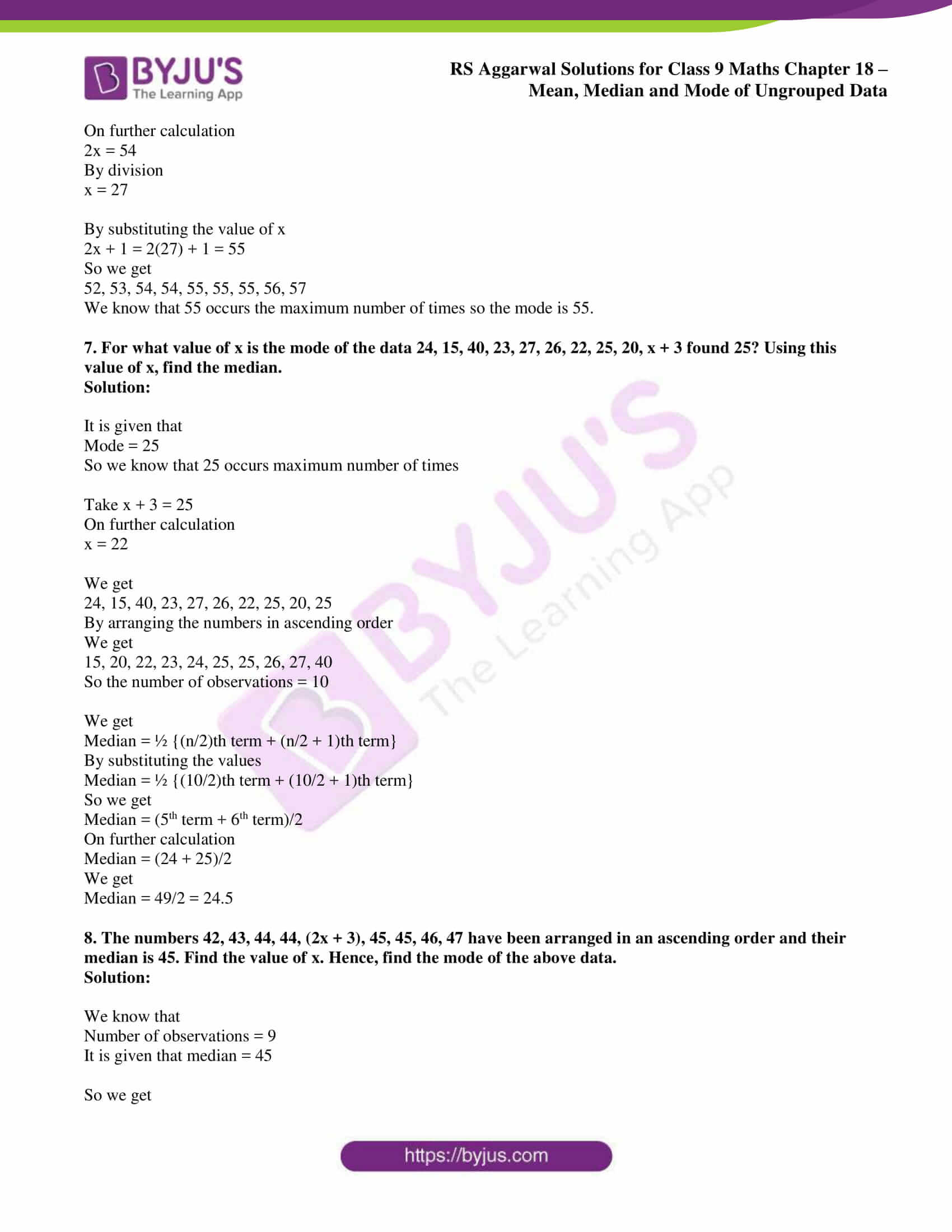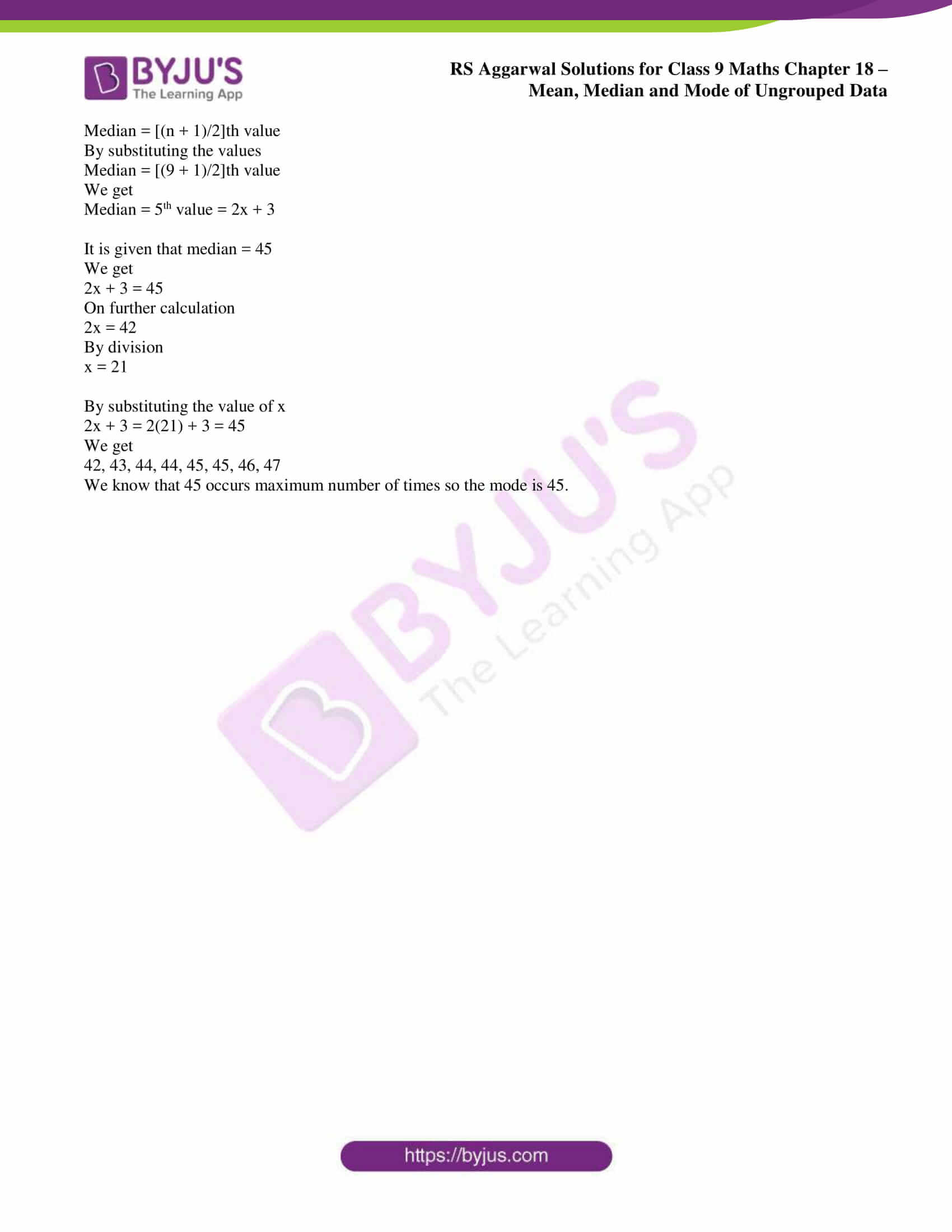# RS Aggarwal Solutions for Class 9 Chapter 18: Mean, Median and Mode of Ungrouped Data Exercise 18D

## RS Aggarwal Solutions for Class 9 Maths Exercise 18D PDF

The solutions are prepared with the aim of helping students perform well in board exams. The PDF of solutions contains detailed exercise wise answers which are designed based on the CBSE guidelines. The solutions prepared by our faculty are designed based on exam pattern of current CBSE board. The students while solving RS Aggarwal textbook exercise problems can use PDF to clear doubts and understand the concepts. RS Aggarwal Solutions for Class 9 Maths Chapter 18 Mean, Median and Mode of Ungrouped Data Exercise 18D are provided here.

## RS Aggarwal Solutions for Class 9 Chapter 18: Mean, Median and Mode of Ungrouped Data Exercise 18D Download PDF## Access RS Aggarwal Solutions for Class 9 Chapter 18: Mean, Median and Mode of Ungrouped Data Exercise 18D

1. Find the mode of the following items.

0, 6, 5, 1, 6, 4, 3, 0, 2, 6, 5, 6

Solution:

By arranging the numbers in ascending order

We get

0, 0, 1, 2, 3, 4, 5, 5, 6, 6, 6, 6

 Observations (x) 0 1 2 3 4 5 6 Frequency 2 1 1 1 1 2 4

From the table we know that 6 occurs maximum number of times so the mode is 6.

2. Determine the mode of the following values of a variable.

23, 15, 25, 40, 27, 25, 22, 25, 20

Solution:

By arranging the numbers in ascending order

We get

15, 20, 22, 23, 25, 25, 25, 27, 40

 Observations (x) 15 20 22 23 25 27 40 Frequency 1 1 1 1 3 1 1

From the table we know that 25 occurs maximum number of times so the mode is 25.

3. Calculate the mode of the following sizes of shoes sold by a shop on a particular day.

5, 9, 8, 6, 9, 4, 3, 9, 1, 6, 3, 9, 7, 1, 2, 5, 9

Solution:

By arranging the numbers in ascending order

We get

1, 1, 2, 3, 3, 4, 5, 5, 6, 6, 7, 8, 9, 9, 9, 9, 9

 Observations (x) 1 2 3 4 5 6 7 8 9 Frequency 2 1 2 1 2 2 1 1 5

From the table we know that 9 occurs maximum number of times so the mode is 9.

4. A cricket player scored the following runs in 12 one-day matches:

50, 30, 9, 32, 60, 50, 28, 50, 19, 50, 27, 35.

Find his modal score.

Solution:

By arranging the numbers in ascending order

We get

9, 19, 27, 28, 30, 32, 35, 50, 50, 50, 50, 60

 Observations (x) 9 19 27 28 30 32 35 50 60 Frequency 1 1 1 1 1 1 1 4 1

From the table we know that 50 occurs maximum number of times so the mode is 50.

5. If the mean of the data 3, 21, 25, 17, (x + 3), 19, (x – 4) is 18, find the value of x. Using this value of x, find the mode of the data.

Solution:

We know that

Number of observations = 7

It is given that mean = 18

It can be written as

(3 + 21 + 25 + 17 + x + 3 + 19 + x – 4)/7 = 18

On further calculation

2x + 84 = 126

By subtraction

2x = 42

By division

x = 21

By substituting the value of x

(x + 3) = 21 + 3 = 24

(x – 4) = 21 – 4 = 17

So we get

3, 21, 25, 17, 24, 19, 17

We know that 17 occurs maximum number of times so the mode is 17.

6. The numbers 52, 53, 54, 54, (2x + 1), 55, 55, 56, 57 have been arranged in an ascending order and their median is 55. Find the value of x and hence find the mode of the given data.

Solution:

We know that

Number of observations = 9

By arranging the numbers in ascending order

We get

52, 53, 54, 54, (2x + 1), 55, 55, 56, 57

So we get

Median = [(n + 1)/2]th value

By substituting the values

Median = [(9 + 1)/2]th value

We get

Median = 5th value = 2x + 1

It is given that median = 55

We can write it as

2x + 1 = 55

On further calculation

2x = 54

By division

x = 27

By substituting the value of x

2x + 1 = 2(27) + 1 = 55

So we get

52, 53, 54, 54, 55, 55, 55, 56, 57

We know that 55 occurs the maximum number of times so the mode is 55.

7. For what value of x is the mode of the data 24, 15, 40, 23, 27, 26, 22, 25, 20, x + 3 found 25? Using this value of x, find the median.

Solution:

It is given that

Mode = 25

So we know that 25 occurs maximum number of times

Take x + 3 = 25

On further calculation

x = 22

We get

24, 15, 40, 23, 27, 26, 22, 25, 20, 25

By arranging the numbers in ascending order

We get

15, 20, 22, 23, 24, 25, 25, 26, 27, 40

So the number of observations = 10

We get

Median = ½ {(n/2)th term + (n/2 + 1)th term}

By substituting the values

Median = ½ {(10/2)th term + (10/2 + 1)th term}

So we get

Median = (5th term + 6th term)/2

On further calculation

Median = (24 + 25)/2

We get

Median = 49/2 = 24.5

8. The numbers 42, 43, 44, 44, (2x + 3), 45, 45, 46, 47 have been arranged in an ascending order and their median is 45. Find the value of x. Hence, find the mode of the above data.

Solution:

We know that

Number of observations = 9

It is given that median = 45

So we get

Median = [(n + 1)/2]th value

By substituting the values

Median = [(9 + 1)/2]th value

We get

Median = 5th value = 2x + 3

It is given that median = 45

We get

2x + 3 = 45

On further calculation

2x = 42

By division

x = 21

By substituting the value of x

2x + 3 = 2(21) + 3 = 45

We get

42, 43, 44, 44, 45, 45, 46, 47

We know that 45 occurs maximum number of times so the mode is 45.

### Access other exercise solutions of Class 9 Maths Chapter 18: Mean, Median and Mode of Ungrouped Data

Exercise 18A Solutions 31 Questions

Exercise 18B Solutions 14 Questions

Exercise 18C Solutions 10 Questions

### RS Aggarwal Solutions Class 9 Maths Chapter 18 – Mean, Median and Mode of Ungrouped Data Exercise 18D

RS Aggarwal Solutions Class 9 Maths Chapter 18 Mean, Median and Mode of Ungrouped Data Exercise 18D contains answers to problems which are based on finding the mode of ungrouped data.

### Key features of RS Aggarwal Solutions for Class 9 Maths Chapter 18: Mean, Median and Mode of Ungrouped Data Exercise 18D

• The PDF of solutions help in inculcating right attitude to studies among the students.
• The solutions are explained in such a way that they make the important concepts of each chapter clear.
• Using the solutions improves efficiency in solving exercise wise problems of RS Aggarwal textbook.
• The students can solve the questions and practice them at their own pace with a better understanding of concepts.